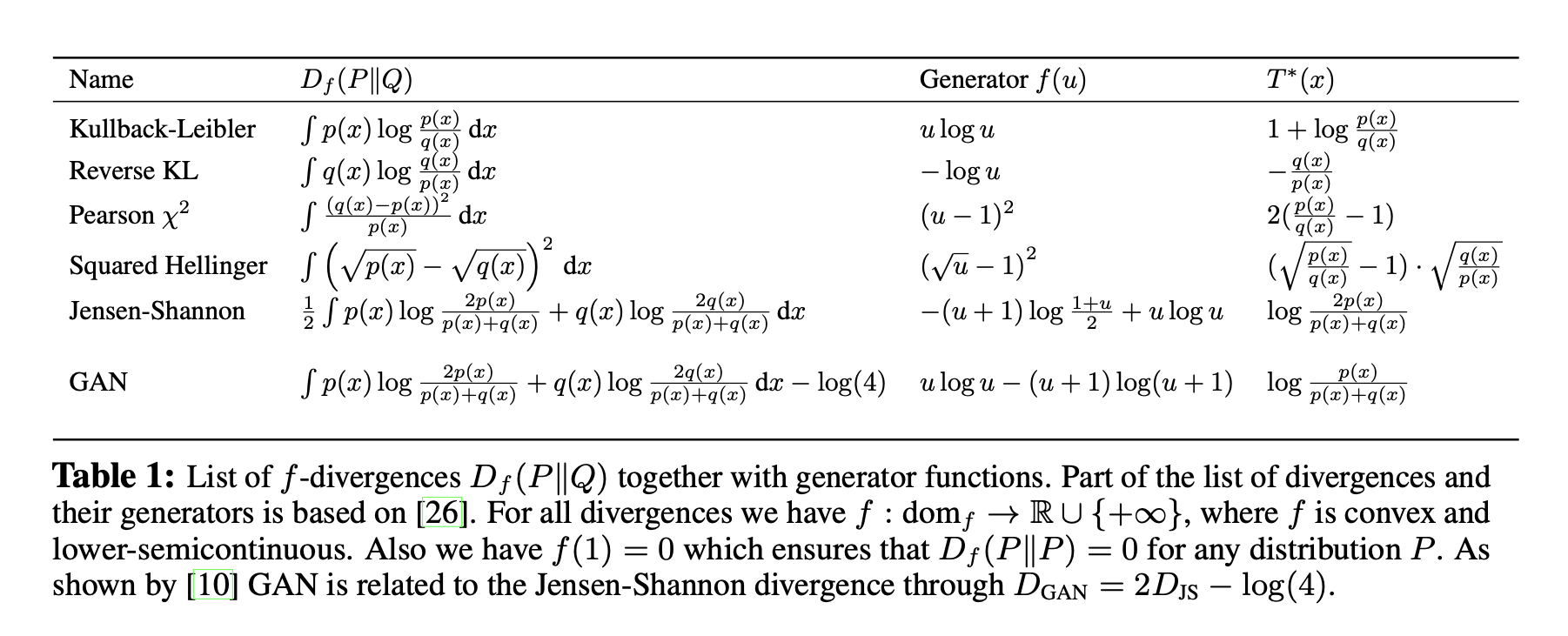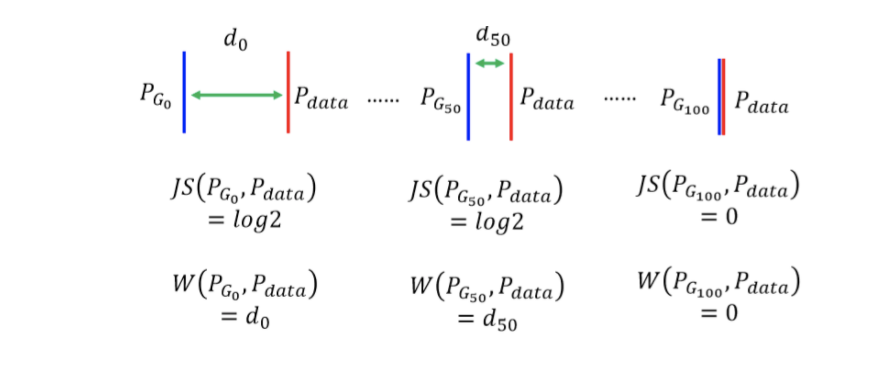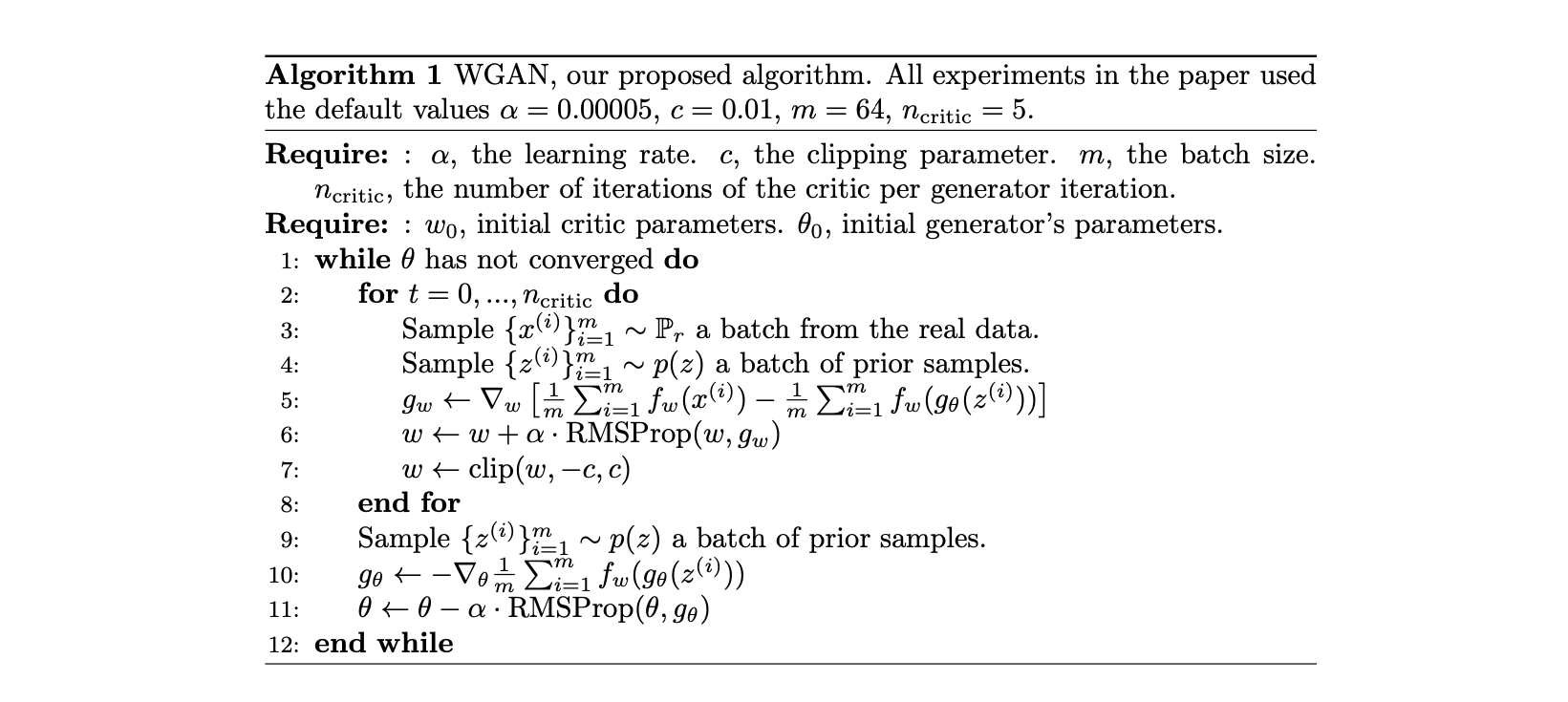N1H111SM's Miniverse

2020/06/16 ShareMaterials

# Vanilla GAN

Deep Learning研究的问题本质上是希望学习从一个数据空间到另一个数据空间的映射；更准确地，一个distribution向另一个distribution的映射。在分类任务中，这两个分布分别为合法数据点的分布向0-1分布的映射；在回归任务中，这两个分布分别为特征空间内数据点的分布向预测空间内的分布。

GAN的核心思想在于将寻找最优generator $G$ 的过程刻画成一个由generator和discriminator两者构成的minmax game：

where

# f-GAN

Vanilla GAN的优化目标是JS divergence $\operatorname{JS}(P_{data} | P_G)$, 而f-GAN这篇工作则把满足一定特性的不同divergence都归在了同一个f-divergence的框架下，并且提出了对应在minmax game中优化函数的表达式。

## The f-divergence Family

A large class of different divergences are the so called f-divergences, also known as the Ali-Silvey distances. Given two distributions $P$ and $Q$ that possess, respectively, an absolutely continuous density function $p$ and $q$ with respect to a base measure $dx$ defined on the domain $\mathcal{X}$ , we define the f-divergence:

where the generator function $f: \mathbb R_+ \rightarrow \mathbb R$ is a convex, lower-semicontinuous function satisfying $f (1) = 0$.

## Estimating f-divergence

Since $f$ is convex, we have

Definition (Jensen Inequality). If $X$ is a random variable and $\varphi$ is a convex function, then following inequality holds.

where $t=T(x)​$, $T: \mathcal{X} \rightarrow \mathbb{R}​$. 接下来我们推导原文中略过的部分：在Legendre Transformation的语境下我们可以求出相对紧的下界 $T^*​$ (注意不要和conjugate的符号混淆).## Variational Divergence Minimization (VDM)

To this end, we follow the generative-adversarial approach and use two neural networks, $Q$ and $T$ . $Q$ is our generative model, taking as input a random vector and outputting a sample of interest. We parametrize $Q$ through a vector $\theta$ and write $Q_\theta$. $T$ is our variational function, taking as input a sample and returning a scalar. We parametrize $T$ using a vector ω and write $T_\omega$ .

# WGAN

WGAN使用了在f-GAN family 之外的用于衡量两个分布的距离函数: Earth-Mover (EM) distance or Wasserstein-1.

Definition (Earth-Mover Distance). The Earth-Mover distance between two distribution $\mathbb P_r$ and $\mathbb P_g$ is defined as

where $\Pi (\mathbb P_r, \mathbb P_g)$ denotes the set of all joint distributions $\gamma (x,y)$ whose marginals are respectively $\mathbb P_r$ and $\mathbb P_g$. Intuitively, $\gamma (x,y)$ indicates how much “mass” must be transported from $x$ to $y$ in order to transform the distributions $\mathbb P_r$ into the distribution $\mathbb P_g$. The EM distance then is the “cost” of the optimal transport plan.WGAN需要对参数化的函数需要进行限制：那就是将函数限制在K-Lipschitz的function family中。文章的方法就是做weight clipping. 当然这个方法导致的具体的$K$是多少，我们就没有办法得知了。

In order to have parameters $W$ lie in a compact space, something simple we can do is clamp the weights to a fixed box (say $W = [−0.01, 0.01]^l$) after each gradient update.